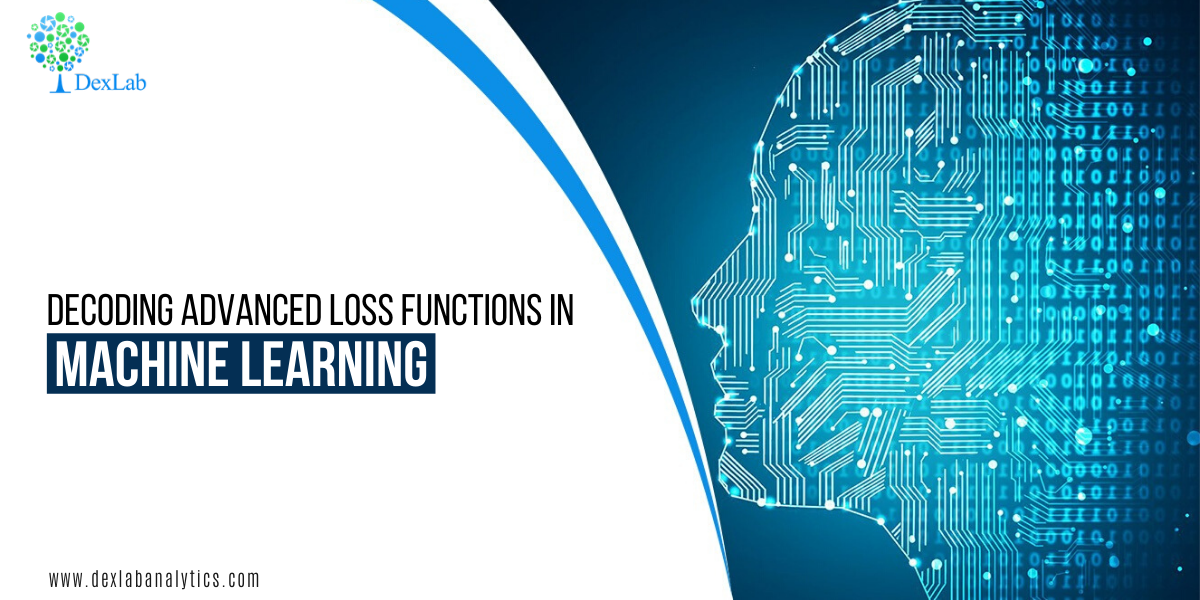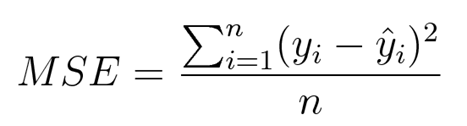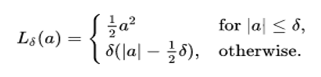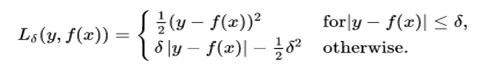# Decoding Advanced Loss Functions in Machine Learning: A Comprehensive GuideEvery Machine Learning algorithm (Model) learns by the process of optimizing the loss functions. The loss function is a method of evaluating how accurate the given prediction is made. If predictions are off, then loss function will output a higher number. If they’re pretty good, it’ll output a lower number. If someone makes changes in the algorithm to improve the model, loss function will show the path in which one should proceed.

Machine Learning is growing as fast as ever in the age we are living, with a host of comprehensive Machine Learning course in India pacing their way to usher the future. Along with this, a wide range of courses like Machine Learning Using Python, Neural Network Machine Learning Python is becoming easily accessible to the masses with the help of Machine Learning institute in Gurgaon and similar institutes.We are having different types of loss functions.

• Regression Loss Functions
• Binary Classification Loss Functions
• Multi-class Classification Loss Functions

#### Regression Loss Functions

1. Mean Squared Error
2. Mean Absolute Error
3. Huber Loss Function

#### Binary Classification Loss Functions

1. Binary Cross-Entropy
2. Hinge Loss

#### Multi-class Classification Loss Functions

1. Multi-class Cross Entropy Loss
2. Kullback Leibler Divergence Loss

#### Mean Squared Error

Mean squared error is used to measure the average of the squared difference between predictions and actual observations. It considers the average magnitude of error irrespective of their direction.This expression can be defined as the mean value of the squared deviations of the predicted values from that of true values. Here ‘n’ denotes the total number of samples in the data.

#### Mean Absolute Error

Absolute Error for each training example is the distance between the predicted and the actual values, irrespective of the sign.

### MAE = | y-f(x) |

Absolute Error is also known as the L1 loss. The MAE cost is more robust to outliers as compared to MSE.

#### Huber Loss

Huber loss is a loss function used in robust regression. This is less sensitive to outliers in data than the squared error loss. The Huber loss function describes the penalty incurred by an estimation procedure f. Huber (1964) defines the loss function piecewise by:This function is quadratic for small values of a, and linear for large values, with equal values and slopes of the different sections at the two points where |a|= 𝛿. The variable “a” often refers to the residuals, that is to the difference between the observed and predicted values a=y-f(x), so the former can be expanded to: –#### Binary Classification Loss Functions

Binary classifications are those predictive modelling problems where examples are assigned one of two labels.

#### Binary Cross-Entropy

Cross-Entropy is the loss function used for binary classification problems. It is intended for use with binary classification.

Mathematically, it is the preferred loss function under the inference framework of maximum likelihood. Cross-entropy will calculate a score that summarizes the average difference between the actual and predicted probability distributions for predicting class 1. The score is minimized and a perfect cross-entropy value is 0.

#### Hinge Loss

The hinge loss function is popular with Support Vector Machines (SVMs). These are used for training the classifiers,

### l(y) = max(0, 1- t•y)

where ‘t’ is the intended output and ‘y’ is the classifier score.

Hinge loss is convex function but is not differentiable which reduces its options for minimizing with few methods.

#### Multi-Class Classification Loss Functions

Multi-Class classifications are those predictive modelling problems where examples are assigned one of more than two classes.

#### Multi-Class Cross-Entropy

Cross-Entropy is the loss function used for multi-class classification problems. It is intended for use with multi-class classification.

Mathematically, it is the preferred loss function under the inference framework of maximum likelihood. Cross-entropy will calculate a score that summarizes the average difference between the actual and predicted probability distributions for all classes. The score is minimized and a perfect cross-entropy value is 0.

#### Kullback Leibler Divergence Loss

KL divergence is a natural way to measure the difference between two probability distributions.

A KL divergence loss of 0 suggests the distributions are identical. In practice, the behaviour of KL Divergence is very similar to cross-entropy. It calculates how much information is lost (in terms of bits) if the predicted probability distribution is used to approximate the desired target probability distribution.

There are also some advanced loss functions for machine learning models which are used for specific purposes.

1. Robust Bi-Tempered Logistic Loss based on Bregman Divergences
2. Minimax loss for GANs
3. Focal Loss for Dense Object Detection
4. Intersection over Union (IoU)-balanced Loss Functions for Single-stage Object Detection
5. Boundary loss for highly unbalanced segmentation
6. Perceptual Loss Function

#### Robust Bi-Tempered Logistic Loss based on Bregman Divergences

In this loss function, we introduce a temperature into the exponential function and replace the softmax output layer of the neural networks by a high-temperature generalization. Similarly, the logarithm in the loss we use for training is replaced by a low-temperature logarithm. By tuning the two temperatures, we create loss functions that are non-convex already in the single-layer case. When replacing the last layer of the neural networks by our bi-temperature generalization of the logistic loss, the training becomes more robust to noise. We visualize the effect of tuning the two temperatures in a simple setting and show the efficacy of our method on large datasets. Our methodology is based on Bregman divergences and is superior to a related two-temperature method that uses the Tsallis divergence.

#### Minimax loss for GANs

Minimax GAN loss refers to the minimax simultaneous optimization of the discriminator and generator models.

Minimax refers to an optimization strategy in two-player turn-based games for minimizing the loss or cost for the worst case of the other player.

For the GAN, the generator and discriminator are the two players and take turns involving updates to their model weights. The min and max refer to the minimization of the generator loss and the maximization of the discriminator’s loss.

#### Focal Loss for Dense Object Detection

The Focal Loss is designed to address the one-stage object detection scenario in which there is an extreme imbalance between foreground and background classes during training (e.g., 1:1000). Therefore, the classifier gets more negative samples (or more easy training samples to be more specific) compared to positive samples, thereby causing more biased learning.

The large class imbalance encountered during the training of dense detectors overwhelms the cross-entropy loss. Easily classified negatives comprise the majority of the loss and dominate the gradient. While the weighting factor (alpha) balances the importance of positive/negative examples, it does not differentiate between easy/hard examples. Instead, we propose to reshape the loss function to down-weight easy examples and thus, focus training on hard negatives. More formally, we propose to add a modulating factor (1 − pt) γ to the cross-entropy loss, with tunable focusing parameter γ ≥ 0.

We define the focal loss as

### FL(pt) = −(1 − pt) γ log(pt)

#### Intersection over Union (IoU)-balanced Loss Functions for Single-stage Object Detection

The IoU-balanced classification loss focuses on positive scenarios with high IoU can increase the correlation between classification and the task of localization. The loss aims at decreasing the gradient of the examples with low IoU and increasing the gradient of examples with high IoU. This increases the localization accuracy of models.

#### Boundary loss for highly unbalanced segmentation

Boundary loss takes the form of a distance metric on the space of contours (or shapes), not regions. This can mitigate the difficulties of regional losses in the context of highly unbalanced segmentation problems because it uses integrals over the boundary (interface) between regions instead of unbalanced integrals over regions. Furthermore, a boundary loss provides information that is complementary to regional losses. Unfortunately, it is not straightforward to represent the boundary points corresponding to the regional softmax outputs of a CNN. Our boundary loss is inspired by discrete (graph-based) optimization techniques for computing gradient flows of curve evolution.

Following an integral approach for computing boundary variations, we express a non-symmetric L2L2 distance on the space of shapes as a regional integral, which avoids completely local differential computations involving contour points. This yields a boundary loss expressed with the regional softmax probability outputs of the network, which can be easily combined with standard regional losses and implemented with any existing deep network architecture for N-D segmentation. We report comprehensive evaluations on two benchmark datasets corresponding to difficult, highly unbalanced problems: the ischemic stroke lesion (ISLES) and white matter hyperintensities (WMH). Used in conjunction with the region-based generalized Dice loss (GDL), our boundary loss improves performance significantly compared to GDL alone, reaching up to 8% improvement in Dice score and 10% improvement in Hausdorff score. It also yielded a more stable learning process.

#### Perceptual Loss Function

We consider image transformation problems, where an input image is transformed into an output image. Recent methods for such problems typically train feed-forward convolutional neural networks using a \emph{per-pixel} loss between the output and ground-truth images. Parallel work has shown that high-quality images can be generated by defining and optimizing \emph{perceptual} loss functions based on high-level features extracted from pre-trained networks. We combine the benefits of both approaches and propose the use of perceptual loss functions for training feed-forward networks for image transformation tasks. We show results on image style transfer, where a feed-forward network is trained to solve the optimization problem proposed by Gatys et al in real-time. Compared to the optimization-based method, our network gives similar qualitative results but is three orders of magnitude faster. We also experiment with single-image super-resolution, where replacing a per-pixel loss with a perceptual loss gives visually pleasing results.

#### Conclusion

Loss function takes the algorithm from theoretical to practical and transforms neural networks from matrix multiplication into deep learning. In this article, initially, we understood how loss functions work and then, we went on to explore a comprehensive list of loss functions also we have seen the very recent — advanced loss functions.

References: –

https://arxiv.org
https://www.wikipedia.org

#### Interested in a career in Data Analyst?### Call us to know more### Gurgaon+91 931 572 5902